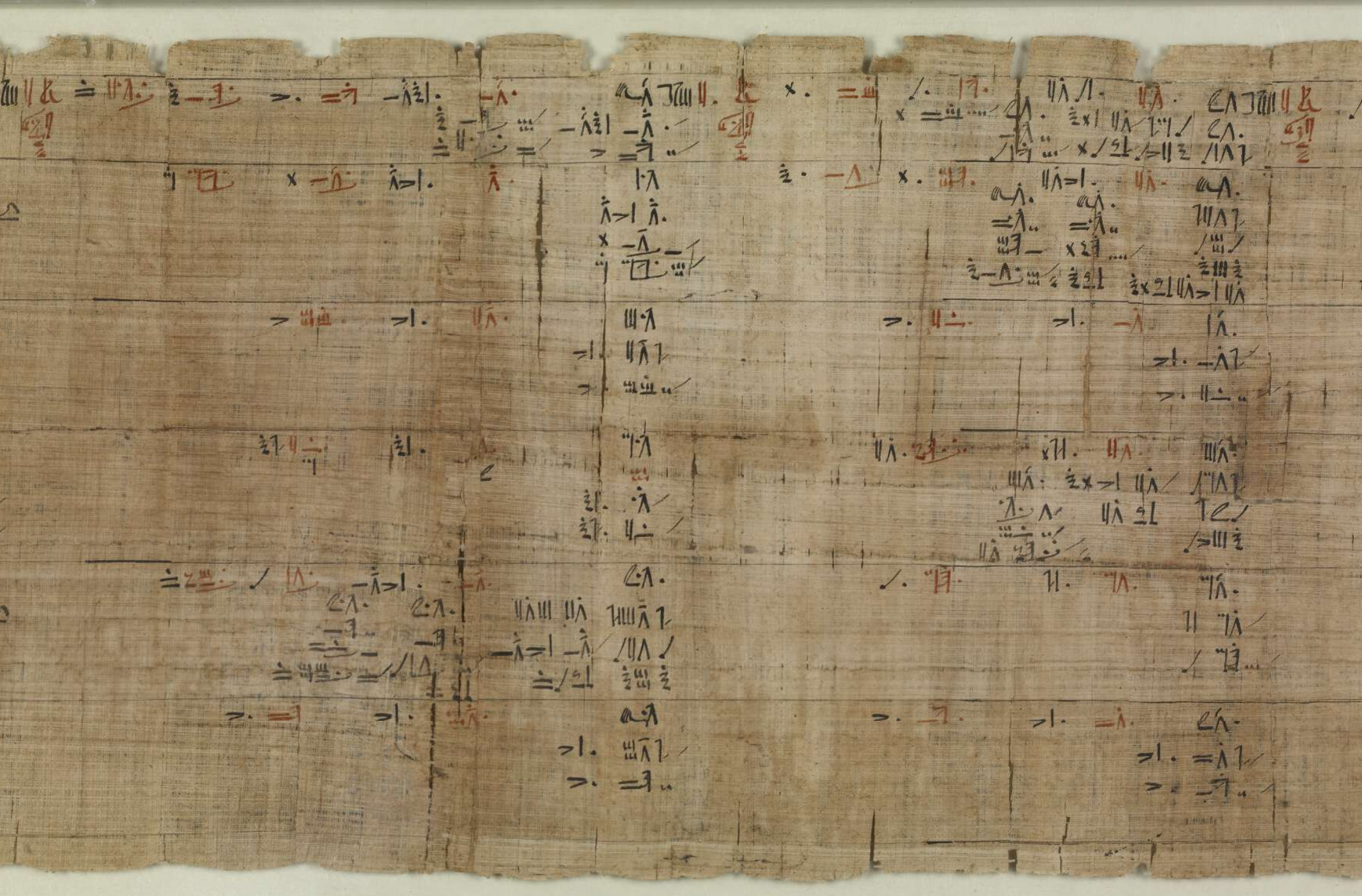## Welcome to MAT 336 - History of Mathematics

Lectures 1 and 2 - Fall 2021

This is , in the opinion of your instructor, a fascinating course about how we - human beings- created and developed mathematical ideas. It will allow us to think about these mathematical ideas and look at how different groups develop them with their own point of view.### A portion of the Rhind Mathematical Papirus

Possibly a math textbook.

## The syllabus can be found here in PDF, four slides per page, and here, one slide per page.

Addition to the syllabus: A "math point" is a concrete purely mathematical aspect of the topic which is mastered by the student. This math point can be, for instance, the solution of a problem, or the proof of a statement. It does not have to be the "whole" mathematical aspect of the topic. Examples of math point are:

• Combinations and binomial coefficients as explained in Pascal’s "Traite du triangle arithmetique".
• Liu Hui and Tsu Keng-chih 's calculation of the Volume of a Sphere
• Analogues of Pythagorean Theorem with the areas of different shapes, for instance squares, triangles and trapezoids.
• Desargues Theorem